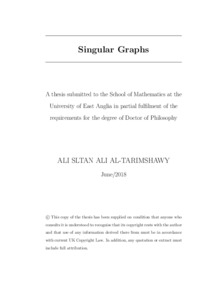# Singular graphs

AL-Tarimshawy, Ali Sltan Ali (2018) Singular graphs. Doctoral thesis, University of East Anglia.Preview
PDF
Download (559kB) | Preview

## Abstract

Let Γ be a simple graph on a ﬁnite vertex set V and let A be its adjacency matrix. Then Γ is said to be singular if and only if 0 is an eigenvalue of A. The nullity (singularity) of Γ, denoted by null(Γ), is the algebraic multiplicity of the eigenvalue 0 in the spectrum of Γ. In 1957, Collatz and Sinogowitz  posed the problem of characterizing singular graphs. Singular graphs have important applications in mathematics and science. In chemistry the importance of singular graphs lies in the fact that a singular molecular graph, with vertices formed by atoms, edges corresponding to bonds between the atoms in the molecule, often is associated to compounds that are more reactive or unstable. By this reason, the chemists have a great interest in this problem. The general problem of characterising singular graphs is easy to state but it seems too diﬃcult at this time. In this work, we investigate this problem for graphs in general and graphs with a vertex transitive group G of automorphisms. In some cases we determine the nullity of such graphs. We characterize singular Cayley graphs over cyclic groups. We show that vertex transitive graphs where |V| is prime are non-singular. The relationship between the irreducible representations of G and the eigenspaces of Γ is studied.

Item Type: Thesis (Doctoral) Faculty of Science > School of Mathematics Jackie Webb 15 Nov 2018 14:27 15 Nov 2018 14:27 https://ueaeprints.uea.ac.uk/id/eprint/68916

### Actions (login required)

 View Item